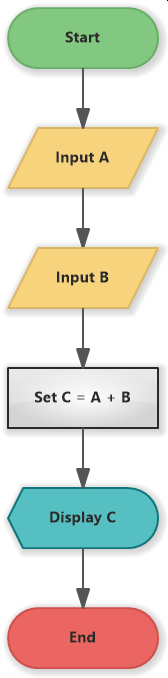# Addition of Two Numbers (Flowchart)

This simple flowchart example shows an addition of two numbers.

The flowchart depicts the algorithm for the addition of two given numbers. The user inputs number A and B, the program calculates their sum and sets the result to the variable C, then it displays the variable C with the result.

The flow is very simple, with a finite number of steps and any conditions.

## Flowchart